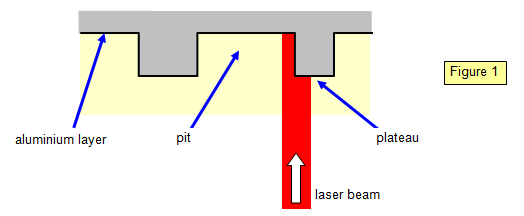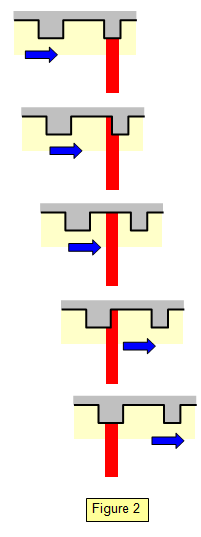Reading a CD using a laser beam

The following set of diagrams explains how a laser beam is used to 'read' a CD. The digital output, zeros and ones, results from the interference at the surface of the aluminium layer.In the condition shown in the large diagram (Figure 1) part of the laser beam falls on a plateau and part on a pit. The pit is one quarter of a wavelength deep and so destructive interference results giving a zero. The following set of small diagrams (Figure 2) shows the change from zero to one in the reflected intensity.Laser beam reflected from a plateau ñ constructive interference results and so the output signal is high ñ a one.

Laser beam reflected from the edge of a plateau ñ destructive interference results and so the output signal is low ñ a zero.

Laser beam reflected from a pit ñ constructive interference results and so the output signal is high ñ a one.

Laser beam reflected from the edge of a plateau ñ destructive interference results and so the output signal is low ñ a zero.

Laser beam reflected from a plateau ñ constructive interference results and so the output signal is high ñ a one.

A VERSION IN WORD IS AVAILABLE ON THE SCHOOLPHYSICS USB